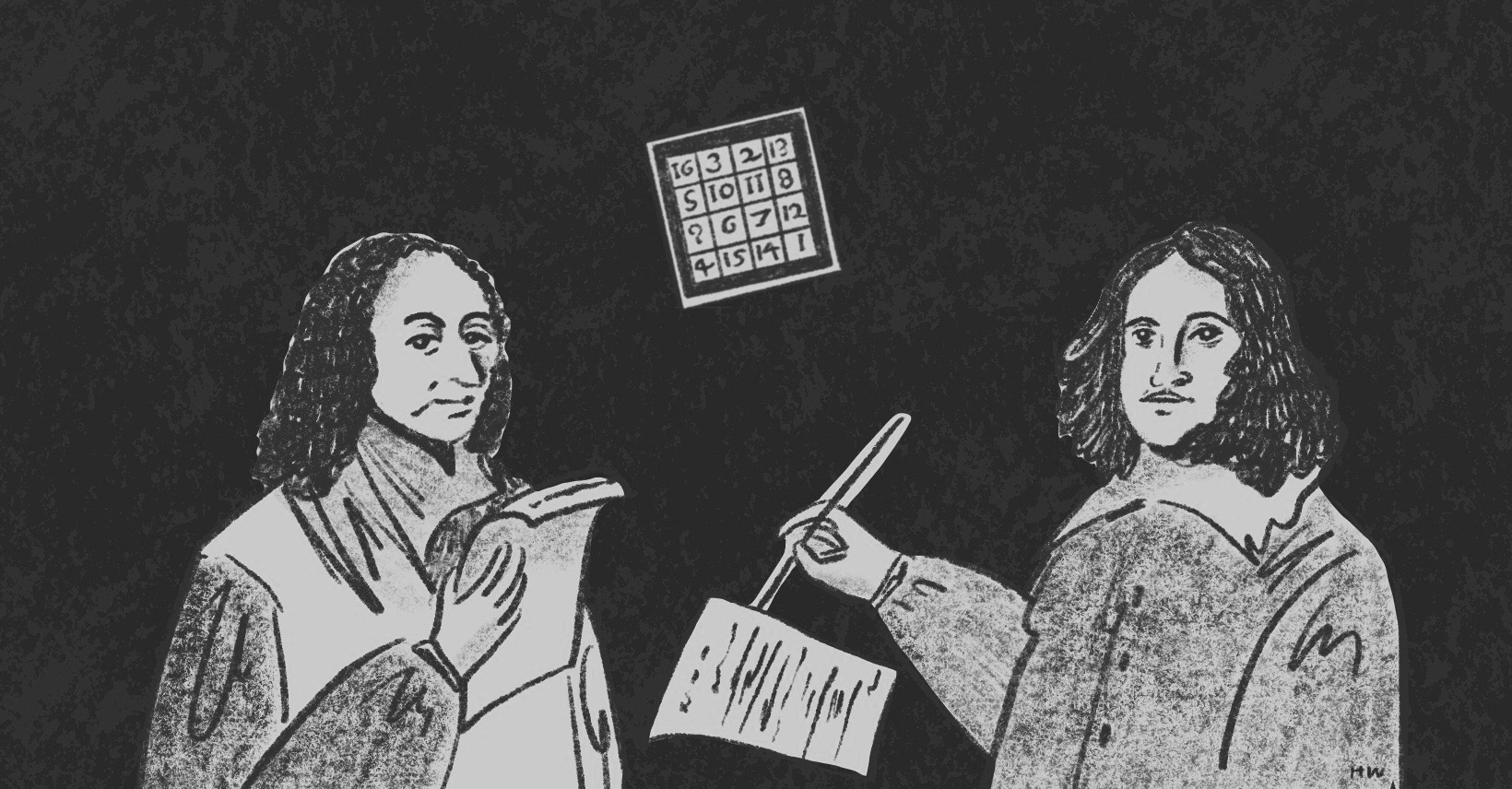×資料管理 統計分析 相關資源 巨人肩膀 語法索引 關於作者

## 簡介

• cohen.ES() -計算效果量數值
• cohens_w() -計算Cohen's w
• pwr.chisq.test() -卡方檢定力
• pwr.r.test() -相關分析檢定力
• cohens_d() -計算Cohen's d
• pwr.t.test() -平均數分析檢定力
• cohens_f() -計算Cohen's f
• pwr.anova.test() -變異數分析檢定力
• cohens_f_squared -計算Cohen's f2
• pwr.f2.test() -線性回歸檢定力

H0為真

H1為真

## 什麼是檢定力1-$$\beta$$？

$$\beta$$是犯型二錯誤的機率，檢定力1-$$\beta$$是正確判斷的機率，數字背後代表的意義是，在樣本真的有差異的情況下有1-$$\beta$$的機率會統計顯著，$$\beta$$的機率不會顯著。所以檢定力簡單來說是評估一個統計方法有多大power的指標。一個好的統計檢定就是要設法讓$$\alpha$$越小、1-$$\beta$$越大。

## 效果量該怎麼算？

### $$\text{Cohen's } w=\sqrt{\displaystyle\sum_{i=1}^m \frac{(p_{1i}-p_{0i})^2}{p_{0i}}}$$

Cohen's w用於卡方分析。其中m是儲存格的數目，p0i是H0也就是每一個儲存格的期望值，p1i則是H1每一個儲存格的觀察值。

### $$\text{Cohen's }d=\frac{\overline{x_{1}}-\overline{x_{2}}}{s}$$

Cohen's d用於t檢定平均數分析。其中s是兩個變數的合併標準差(pooled standard deviation)。

### $$\text{Cohen's }f=\sqrt{\frac{R^2}{1-R^2}}=\sqrt{\frac{\eta^2}{1-\eta^2}}$$

Cohen's f用於變異數分析。其中$$R^2$$其實就是回歸分析中的判定係數，$$\eta^2=\frac{組間平方和SS_{B}}{總平方和SS_{T}}$$。

### $$\text{Cohen's }f^2=\frac{R^2}{1-R^2}=\frac{\eta^2}{1-\eta^2}$$

Cohen's f2用於線性回歸。其中$$R^2$$其實就是回歸分析中的判定係數。

 > cohen.ES(test = c("p", "t", "r", "anov", "chisq", "f2"),size = c("small", "medium", "large")) 

0.1 0.1 0.2 0.1 0.02
0.3 0.3 0.5 0.25 0.15
0.5 0.5 0.8 0.4 0.35

## 卡方分析的檢定力

pwr.chisq.test()用於分析卡方分析的檢定力，其指令為：

 > pwr.chisq.test(w = NULL, N = NULL, df = NULL, sig.level = 0.05, power = NULL) 

 > thirdlight_tab<-matrix(c(6773, 22959, 7161, 25989), ncol=2, byrow=TRUE) > colnames(thirdlight_tab)<-c("Rear Impacts", "Others") > rownames(thirdlight_tab)<-c("1985 (without)", "1986 (equipped)") > library(effectsize) > cohens_w(thirdlight_tab) Phi | 95% CI ------------------- 0.01 | [0.01, 1.00] - One-sided CIs: upper bound fixed at (1). 

 > pwr.chisq.test(w=0.01, N=62882, df=1, sig.level=0.05) Chi squared power calculation w = 0.01 N = 62882 df = 1 sig.level = 0.05 power = 0.7080428 NOTE: N is the number of observations 

 > pwr.chisq.test(w=0.01, power=0.71, df=1, sig.level=0.05) Chi squared power calculation w = 0.01 N = 63168.65 df = 1 sig.level = 0.05 power = 0.71 NOTE: N is the number of observations 

## 相關分析的檢定力

pwr.r.test()用於相關係數的檢定力分析，其指令為：

 > pwr.r.test(n = NULL, r = NULL, sig.level = 0.05, power = NULL, alternative = c("two.sided", "less","greater")) 

 > pwr.r.test(n=31, r=0.6406726, sig.level = 0.05) approximate correlation power calculation (arctangh transformation) n = 31 r = 0.6406726 sig.level = 0.05 power = 0.9825784 alternative = two.sided 

 > pwr.r.test(r=0.6406726, sig.level = 0.05, power=0.8) approximate correlation power calculation (arctangh transformation) n = 15.94704 r = 0.6406726 sig.level = 0.05 power = 0.8 alternative = two.sided 

## 平均數分析的檢定力

pwr.t.test()是pwr中處理獨立樣本、成對樣本t檢定的檢定力分析指令。如果兩個樣本數不同則可改用pwr.t2n.test()。其指令為：

 > pwr.t.test(n = NULL, d = NULL, sig.level = 0.05, power = NULL, type = c("two.sample", "one.sample", "paired"), alternative = c("two.sided", "less", "greater")) > pwr.t2n.test(n1 = NULL, n2 = NULL, d = NULL, sig.level = 0.05, power = NULL, alternative = c("two.sided", "less", "greater")) 

 > trad_med<-read.csv("c:/Users/USER/downloads/gender_traditionalmed.csv", header=T, sep=",") > cohens_d(trad_med$Female, trad_med$Male) Cohen's d | 95% CI ------------------- 5.29 | [4.01, 6.56] - Estimated using pooled SD. 

 > pwr.t.test(n=22, d=5.29, sig.level=0.05) Two-sample t test power calculation n = 22 d = 1 sig.level = 0.05 power = 1 alternative = two.sided NOTE: n is number in *each* group 

 > pwr.t.test(d=0.8, power=0.8, sig.level = 0.05) approximate correlation power calculation (arctangh transformation) n = 2.060225 d = 5.29 sig.level = 0.05 power = 0.8 alternative = two.sided NOTE: n is number in *each* group 

## 變異數分析的檢定力

pwr處理變異數分析的檢定力指令是pwr.anova.test()，其指令為：

 > pwr.anova.test(k = NULL, n = NULL, f = NULL, sig.level = 0.05, power = NULL) 

 > rate<-read.csv("c:/Users/USER/downloads/tv_rate.csv", header=TRUE, sep=",") > oneway<-aov(Rate~Type, data=rate) > cohens_f(oneway) For one-way between subjects designs, partial eta squared is equivalent to eta squared. Returning eta squared. # Effect Size for ANOVA Parameter | Cohen's f | 95% CI ---------------------------------------- Type | 0.68 | [0.27, Inf] - One-sided CIs: upper bound fixed at (Inf). 

 > pwr.anova.test(k=3, n=30, f=0.25, sig.level=0.05) Balanced one-way analysis of variance power calculation k = 3 n = 30 f = 0.68 sig.level = 0.05 power = 0.9999702 NOTE: n is number in each group 

 > pwr.anova.test(k=3, f=0.68, power=0.99, sig.level=0.05) Balanced one-way analysis of variance power calculation k = 3 n = 16.47782 f = 0.68 sig.level = 0.05 power = 0.99 NOTE: n is number in each group 

## 線性回歸的檢定力

pwr.f2.test()是一般線性模型的檢定力指令。這裡的u指的是分子的自由度，v是分母的自由度。根據Cohen的解釋u等於模型中解釋變數的數目，v等於n-u-1。

 > pwr.f2.test(u = NULL, v = NULL, f2 = NULL, sig.level = 0.05, power = NULL) 

 > phillips<-read.csv("c:/Users/USER/downloads/phillips_curve.csv", header=T, sep=",") > model<-lm(wage~unemployment, data=phillips) > cohens_f_squared(model) For one-way between subjects designs, partial eta squared is equivalent to eta squared. Returning eta squared. # Effect Size for ANOVA Parameter | Cohen's f2 | 95% CI -------------------------------------------- unemployment | 1.77 | [0.79, Inf] - One-sided CIs: upper bound fixed at (Inf). 

 > pwr.f2.test(u=1, v=28, f=1.77, sig.level=0.05) Multiple regression power calculation u = 1 v = 28 f2 = 1.77 sig.level = 0.05 power = 0.9999998 

 > pwr.f2.test(u=1, f=0.15, power=0.8, sig.level=0.05) Multiple regression power calculation u = 1 v = 10.71178 f2 = 1.77 sig.level = 0.05 power = 0.99